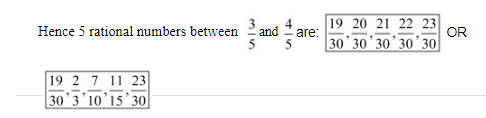# Find five rational numbers between

Question:

Find five rational numbers between $\frac{3}{5}$ and $\frac{4}{5}$.

Solution:

We need to find 5 rational numbers between $\frac{3}{5}$ and $\frac{4}{5}$.

Since, LCM of denominators $=L C M(5,5)=5$

So, consider

$\frac{3}{5}=\frac{3}{5} \times \frac{6}{6}$

$\Rightarrow \frac{3}{5}=\frac{18}{30}$

And,

$\Rightarrow \frac{4}{5}=\frac{4}{5} \times \frac{6}{6}$

$\Rightarrow \frac{4}{5}=\frac{24}{30}$